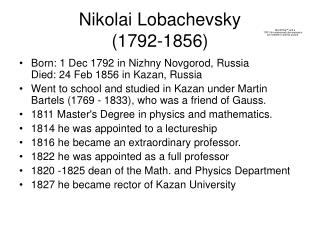Download PresentationNikolai Lobachevsky (1792-1856)

# Nikolai Lobachevsky (1792-1856)

Download Presentation## Nikolai Lobachevsky (1792-1856)

- - - - - - - - - - - - - - - - - - - - - - - - - - - E N D - - - - - - - - - - - - - - - - - - - - - - - - - - -
##### Presentation Transcript

1. Nikolai Lobachevsky (1792-1856) • Born: 1 Dec 1792 in Nizhny Novgorod, RussiaDied: 24 Feb 1856 in Kazan, Russia • Went to school and studied in Kazan under Martin Bartels (1769 - 1833), who was a friend of Gauss. • 1811 Master's Degree in physics and mathematics. • 1814 he was appointed to a lectureship • 1816 he became an extraordinary professor. • 1822 he was appointed as a full professor • 1820 -1825 dean of the Math. and Physics Department • 1827 he became rector of Kazan University

2. Nikolai Lobachevsky (1792-1856) Accepting the possibility of HAA Lobachevsky redefines parallels in the following way: 16. All straight lines which in a plane go out from a point can, with reference to a given straight line in the same plane, be divided into two classes - into cutting and non-cutting. The boundary lines of the one and the other class of those lines will be called parallel to the given line.

3. Nikolai Lobachevsky (1792-1856)

4. Nikolai Lobachevsky (1792-1856) Depending on the angle HAD which is called (p) there are two possibilities. Either • (p)=π/2 and there is only one parallel to CB through A, or • (p)<π/2 there are exactly two Lobachevsky parallels. This means that there are infinitely many lines through A which do not intersect CB.

5. Nikolai Lobachevsky (1792-1856) In the case that there are two parallels there are also two sides of parallelism. l’ The side of parallelism of l’ (p) p (p) The side of parallelism of l’’ l’’

6. Nikolai Lobachevsky (1792-1856) Lobachevsky needs to prove the following. His definition of parallelism uses the point A, he has to show that in fact parallelism is a symmetric property of two lines • 17. A straight line maintains the characteristic of parallelism at all its points. • 18. Two lines are always mutually parallel.

7. Nikolai Lobachevsky (1792-1856) He shows that HOA is not possible with the first four of Euclid’s axioms (which he made more precise e.g. #3). 19. In a rectilineal triangle the sum of the three angles can not be greater than two right angles.

8. Nikolai Lobachevsky (1792-1856) He also shows that if one triangle has the property that the sum of the interior angels is 180°, then all triangles have this property. This is the dichotomy between Euclidean geometry (HRA) and non-Euclidian geometry (HAA). 20. If in any rectilineal triangle the sum of the three angles is equal to two right angles, so is this also the case for every other triangle.

9. Nikolai Lobachevsky (1792-1856) Finally he shows that the dichotomyextends to parallels. • In Euclidean geometry there is exactly one parallel line to a given line through a given point not on that line. • In non-Euclidean geometry there are exactly two parallel lines, in Lobachevsky’s sense, which implies that there are infinitely many lines through the point that do not intersect the given line. 22. If two perpendiculars to the same straight line are parallel to each other then the sum of the three angles in a rectilineal triangle is equal to two right angels.

10. Lobachevsky’s Euclidean and non-Euclidean geometry • Euclidean or plane geometry is the geometry in which the equivalent assumptions • For all lines and points p: (p) = π/2 and equivalently • The sum of the interior angles of any triangle = π hold. • Non-Euclidean (imaginary) geometry is the geometry in which the equivalent assumptions • For all lines and points p: (p)< π/2 and equivalently • The sum of the interior angles of any triangle < π hold.

11. Nikolai Lobachevsky’s Non-Euclidean geometry Now assume that for all lines and points p: (p)< π/2. In this case parallels can have any angle which is less than π/2 and moreover for any given angle there is always a pair of parallels whose angle is that angle 23. For every given angle  there is a line p such that (p)= .

12. Nikolai Lobachevsky’s Non-Euclidean geometry Parallels cease to have the same distance to each other at all points rather: 24. The farther parallel lines are prolonged on the side of their parallelism, the more they approach on another. B x B’ s s’ A’ A x Moreover in the above figure s’=se-x.